# 38 Assigning Names to Things

 38 Assigning Names to Things
Do a simple computation:
Range

% gives the result of the previous computation:
%

This squares the most recent result:
%^2

If you expect to refer to a result later, you can assign it a name. For example, you can say thing=Range to assign thing as a name for the result of Range.
Assign thing to be the result of Range:
thing = Range

Whenever thing appears, it’ll be replaced by the result of Range:
thing

Square the value of thing:
thing^2

You can assign a name to the result of a computation even if you never display the result. End your input with ; (semicolon) to avoid seeing the result.
Assign a name to a list of a million elements, but don’t display the list:
millionlist = Range;
Find the total of all the elements in the list:
Total[millionlist]

Assign x the value 42:
x = 42

You might have thought this would be {x, y, z}but x has value 42:
{x, y, z}

Clear any assignment for x:
Clear[x]
Now x, like y and z, isn’t replaced:
{x, y, z}

Assigning a global value for x, like x=42, is potentially a big deal, because it can affect everything you do in your session (at least until you clear x). Something that’s much saferand extremely usefulis just to assign a temporary, local value to x, inside a module.
This locally sets the value of x to be Range inside the Module:
Module[{x = Range}, x^2]

Outside the module, x still has no value assigned:
x

You can have as many local variables inside a module as you want.
Define local variables x and n, then compute x^n using the values you’ve assigned:
Module[{x = Range, n = 2}, x^n]

To specify sequences of actions in the Wolfram Language one just separates them by semicolons (;). (Putting a semicolon at the end is like specifying an empty final action, which is why this has the effect of not displaying a result.)
Do a sequence of operations; the result is what the last operation produces:
x = Range; y = x^2; y = y + 10000

This defined global values for x and y; don’t forget to clear them:
Clear[x, y]
You can use semicolons to do sequences of operations inside Module.
This does a sequence of operations, with x and y maintained as local variables:
Module[{x, y}, x = Range; y = x^2; y = y + 10000]

You can mix local variables that do and don’t have initial values:
Module[{x, y, n = 2}, x = Range; y = x^n; y = y + 10000]

You can nest moduleswhich is useful if you’re building large programs where you want to isolate different parts of your code.
38.1Use Module to compute x^2+x where x is Range»
Expected output:
 Out[]=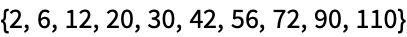Answer & check your solution
38.2Use Module to generate a list of 10 random integers up to 100, then make a column giving the original list, and the results of applying Sort, Max and Total to it. »
Sample expected output:
 Out[]=Answer & check your solution
38.3Use Module to generate an image collage from a picture of a giraffe, and the results of applying Blur, EdgeDetect and ColorNegate to it. »
Sample expected output:
 Out[]=Answer & check your solution
38.4Inside a Module, let r=Range, then make a line plot of r joined with the reverse of r joined with r joined with the reverse of r»
Expected output:
 Out[]=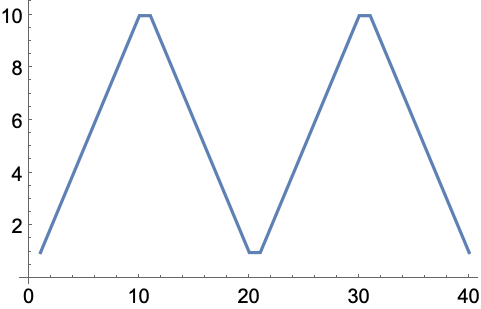Answer & check your solution
38.5Find a simpler form for {Range+1, Range-1, Reverse[Range]}»
Expected output:
 Out[]=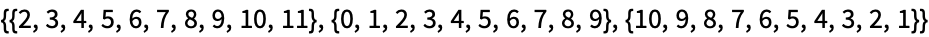Answer & check your solution
38.6Find a simpler form for Module[{u=10}, Join[{u}, Table[u=Mod[17u+2, 11], 20]]]»
Expected output:
 Out[]=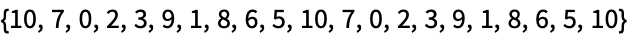Answer & check your solution
38.7Generate 10 random strings made of 5 letters, in which consonants (non-vowels) alternate with vowels (aeiou). »
Sample expected output:
 Out[]=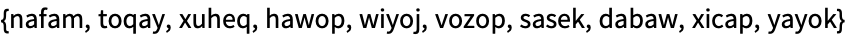Answer & check your solution
How come we’re at Section 38, and only now introducing variable assignments?
Because, as we’ve seen, in the Wolfram Language one can go a very long way without introducing them. And it tends to be a lot cleaner to program without them.
Can I assign a name to anything?
Yes. Graphics, arrays, images, pure functions, whatever.
x equals 4”, or, more rarely, “assign x to 4”, or “give x the value 4”.
What are good principles for global names?
Use names that are specific and explicit. Don’t worry if they’re long; they’ll get autocompleted when you type. For “informal” names, start with lowercase letters. For more carefully thought out names, consider using capitalization like built-in functions do.
% gives the previous result. What about the result before that, etc.?
%% gives the next to last, %%% gives the next-to-next-to-last, etc. %n gives the result on line n (i.e. the result labeled Out[n]).
Can I assign to several variables at once?
Yes. x=y=6 assigns both x and y to 6. {x, y}={3, 4} assigns x to 3 and y to 4. {x, y}={y, x} swaps the values of x and y.
What happens if a variable escapes from a Module without having been assigned a value?
Try it! You’ll find you’ll get a new variable that’s been given a unique name.
What about other procedural programming constructs, like Do and For?
The Wolfram Language has those. Do is sometimes worth using, particularly when your objective is side effects, like assigning variables or exporting data. For is almost always a bad idea, and can almost always be replaced by much cleaner code using constructs such as Table.